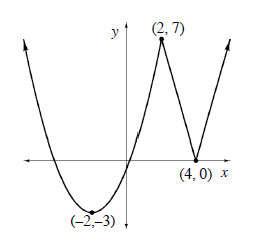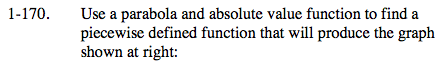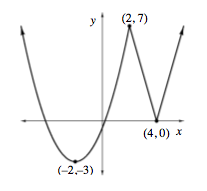### Home > CALC > Chapter 1 > Lesson 1.4.3 > Problem1-170

1-170.
1. Use a parabola and absolute value function to find a piecewise defined function that will produce the graph shown below: Homework Help ✎Vertex form of a parabola: y = a(xh)² + k, where (h, k) is the vertex. Absolute value: y = a|xh| + k

Solve for a by evaluating both equations at (2,7).

Because the function for this graph is piecewise, there is an x-coordinate / boundary point where each function ceases to defined.